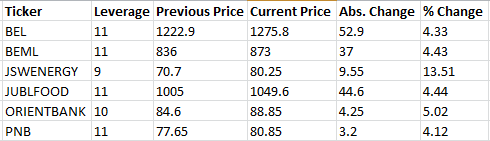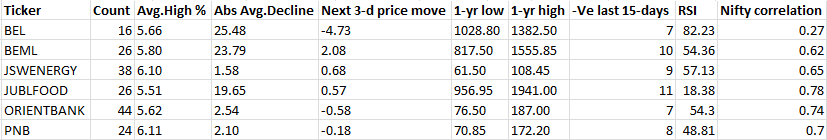# Shorting At High: Algo Trading Strategy In R [EPAT PROJECT]Milind began his career in Gridstone Research, building earnings models and writing earnings notes for NYSE listed companies, covering Technology and REITs sectors. Milind has also worked at CRISIL and Deutsche Bank, where he was involved in modeling of Structured Finance deals covering Asset Backed Securities (ABS), and Collateralized Debt Obligations (CDOs) for the US and EMEA region.

Milind holds a MBA in Finance from the University of Mumbai, and a Bachelor’s degree in Physics from St. Xavier’s College, Mumbai.

### Ideation

The Executive Programme in Algorithmic Trading (EPAT) exposed me to all the requisite subjects needed to learn algorithmic trading. As part of the EPAT project work I tried coding many strategies. Since I am a novice to algorithmic trading I wanted to code the simplest, and the most basic strategies. Although simple and basic, one should not underestimate the power of such strategies, as they can generate good returns.

“Shorting at High” was one of the strategies that I formulated for my project work. This post explains the strategy in brief and the coding part. I welcome the readers to give suggestions, improvise or to use the strategy.

### Strategy in brief

The strategy is to short the best stocks which cross the set percentage threshold on the upside (say 8%-9%) during intraday trading. The expectation for the shorted stocks is to fall by an amount as predicted in the metrics sheet which is generated upon executing the code.

The code works in two parts:

First, we set a high percentage threshold limit in the code. On executing the code, it scans all the stocks in the stock list, and determines which of the stocks have crossed the set threshold limit.

Second, for each of such stocks, it then computes certain metrics like number of times the stock crossed the set threshold in the past one year, average percentage rise during these times, average absolute decline in price for the next three days, RSI, correlation etc. These metrics can then be used to ascertain which stock is the best candidate for taking a short position. The position is squared off at the close or based on one’s profit target.

### Strategy code in R

Step 1: Load the packages, read the stock symbols, and initialize a data frame

First, load the necessary R packages. Set the high threshold level in percentage terms. The next line in the code reads the csv file containing the stocks list. Using this file, we create a vector containing the symbols, and another vector containing the leverage for each stock as provided by the broker.

We also initialize a data frame that will contain basic stock information for each stock after executing the first part of the code. Only those stocks which cross the given threshold will be included in this data frame.

# Upload the required packages

library(xlsx);library(zoo);library(quantmod);library(rowr);library(TTR);

# Set the parameters here

pc_up_level = 6  # Set the high percentage threshold level

noDays = 2       # No of lookback days

# Read the csv file that contains the Stock tickers to be scanned

symbol = as.character(test_tickers[,4])

leverage = as.character(test_tickers[,5])

sig_finds = data.frame(0,0,0,0,0,0)

colnames(sig_finds) = c("Ticker","Leverage","Previous Price","Current Price","Abs Change","% Change")

Step 2: Generating the data frame

If the computed percentage price change is greater than the set threshold, we add that stock to the data frame. For such stocks, the data frame adds information like - the leverage, previous day’s closing price, current price, the absolute change in price, and the percentage change in price.

The resultant data frame after executing the code for all stocks is written to an excel file, which is named as “Shorting at High.xlsx”. All the stock files downloaded from google finance in the R directory are deleted using the unlink command.

for(s in 1:length(symbol)){

print(s)

source("Stock price data.R")

dirPath = paste(getwd(),"/",sep="") # Specify the R directory
fileName = paste(dirPath,symbol[s],".csv",sep="") # Specify the filename

data_close =subset(data, select=(CLOSE), subset=(TIME == 1530))
pdp = tail(data_close,1)[,1]
lp = tail(data,1)[,3]

# Compute absolute and the percentage change
apc = round((lp - pdp),2)
pc = round(((lp - pdp) / pdp) * 100,2)

if (pc > pc_up_level ){

# Create a temporary empty data frame, only if the Percentage change is significant
sig_finds_temp = data.frame(0,0,0,0,0,0)
colnames(sig_finds_temp) = c("Ticker","Leverage","Previous Price","Current Price","Abs Change","% Change")

sig_finds_temp = c(symbol[s],leverage[s],pdp,lp,apc,pc)
sig_finds = rbind(sig_finds, sig_finds_temp) # combines the previous and current data frame

rm(sig_finds_temp) # deletes the temporary data frame

print(sig_finds)

}

# Deletes the downloaded stock price files for each ticker from the directory

} # Write the results in an excel sheet
write.xlsx(sig_finds,"Shorting at High.xlsx")

Example:

Let’s set the threshold at 4%. We have 146 stock symbols in our stock list. When we execute the code, out of the 146 stock symbols, the code has shortlisted 6 stocks which crossed the threshold of 4% during intraday market hours. The table below illustrates the initial output which gets save in the “Shorting at High.xlsx” workbook after executing the code.Step 3: Compute metrics to determine the best stock for shorting

This part of the code uses the stock symbols present in the “Shorting at High.xlsx” sheet. Based on a lookback period of 1 year, the codes in this section compute the following metrics:

• RSI - Relative Strength Index
• Count - The number of times the stock crossed the threshold in the given lookback period
• Avg High % - The average percentage high when the stock went above the threshold
• Abs Avg Decline - The absolute average decline in rupees from the Avg High %
• Next 3-d price move - This gives the absolute price change over the next three days
• Negative last 15-days - No. of times the stock was negative in the last 15-days
• 1-Year High & 1-Year Low for the stock
• Correlation of the stock with NIFTY
# Compute the different metrics which will be used in evaluating the best stock to short # Pull NIFTY data for the last 1 year
source("Stock price data.R")
daily_price_data("NIFTY",1)
dirPath = paste(getwd(),"/",sep="")
fileName = paste(dirPath,"NIFTY",".csv",sep="")
nifty_data = as.data.frame(read.csv(file = fileName))# Read the stock symbols from "Shorting at High.xlsx" workbook
t = nrow(test_tickers)

test_tickers$Count = 0; test_tickers$Avg_high = 0;
test_tickers$Avg_decline = 0; test_tickers$Next_3d_Change = 0;
test_tickers$Low_1Yr = 0; test_tickers$High_1Yr = 0;
test_tickers$Neg_Last15_days = 0 ; test_tickers$RSI = 0;
test_tickers$NIFTY_Correlation = 0 symbol = as.character(test_tickers[2:t,2]) noDays = 1# Compute the metrics for each stock for (s in 1:length(symbol)){ print(s) table_sig = data.frame(0,0,0,0,0,0,0) colnames(table_sig) = c("Date","Days High in %","Days Close in %","RSI","Entry Price","Exit Price","Profit/Loss") source("Stock price data.R") daily_price_data(symbol[s],noDays) dirPath = paste(getwd(),"/",sep="") fileName = paste(dirPath,symbol[s],".csv",sep="") data = as.data.frame(read.csv(file = fileName)) N = nrow(data) # Initializing variables to zero nc = 0 ; Cum_hc_diff = 0; Cum_threshold_per = 0;Cum_next_3d_change = 0; diff = data$HIGH - data$CLOSE rsi_data = RSI(data$CLOSE, n = 14, maType="WMA", wts=data[,"VOLUME"])

for (i in 2:N) {

days_close = (data$CLOSE[i] - data$CLOSE[i-1])*100/data$CLOSE[i-1] days_high = (data$HIGH[i] - data$CLOSE[i-1])*100/data$CLOSE[i-1]

condition = ((days_high > pc_up_level) == TRUE)
if (condition)
{
nc = nc + 1

date_table = data$DATE[i] high_per_table = round(days_high,2) close_price_per_table = format(round(days_close,2),nsmall = 2) rsi_table = format(round(rsi_data[i],2),nsmall = 2) entry_price = format(round(data$CLOSE[i-1]*(1+(pc_up_level/100)),2),nsmall = 2)
exit_price = format(round(data$CLOSE[i],2),nsmall = 2) pl_trade = format(round(((data$CLOSE[i-1]*(1+(pc_up_level/100))) - data$CLOSE[i]),2),nsmall = 2) hc_diff = diff[i] # difference between day's high and day's close whenever the price crossed the threshold level. Cum_hc_diff = Cum_hc_diff + hc_diff # Cumulative of the difference for all trades Average_hc_diff = round(Cum_hc_diff / nc , 2) # Computing the average for difference between high and low. # Computes the total of Day's high's whenever price crossed the threshold. Cum_threshold_per = Cum_threshold_per + days_high # Average of High's Average_threshold_per = round(Cum_threshold_per / nc , 2) if ( i < (N - 5)){ # Captures the average next 3-day price movement next_3d_change = data$CLOSE[i+3] - data$CLOSE[i] Cum_next_3d_change = Cum_next_3d_change + (data$CLOSE[i+3] - data$CLOSE[i]) Average_next_3d_change = round(Cum_next_3d_change / nc,2) } # Create a temporary dataframe to add values and then later merge with the final table table_temp = data.frame(0,0,0,0,0,0,0) colnames(table_temp) = c("Date","Days High in %","Days Close in %","RSI","Entry Price","Exit Price","Profit/Loss") table_temp = c(date_table,high_per_table,close_price_per_table,rsi_table,entry_price,exit_price,pl_trade) table_sig = rbind(table_sig, table_temp) rm(table_temp) } } table_sig = table_sig[order(table_sig$Date),]

# Write the individual results in an excel sheet
name = paste(symbol[s]," past performance",".xlsx",sep="")
write.xlsx(table_sig,name)

# load it back to format the excel sheet for column width
sheets = getSheets(wb)
autoSizeColumn(sheets[], colIndex=1:16)
saveWorkbook(wb,name)

# Price performance in the last 15 days, checks for many days the price change was negative.
nc_last_15 = 0
for ( p in (N-15):N){

condition_1 = (((data$CLOSE[p] - data$CLOSE[p-1])< 0 ) == TRUE )
if (condition_1)
{
nc_last_15 = nc_last_15 + 1
}
}

# Correlation between the ticker and NIFTY
Cor_coeff = round(cor(data$CLOSE , nifty_data$CLOSE),2)

# Filling the “Shorting at High.xlsx” with the computed metrics

e = s + 1
test_tickers$Count[e] = nc test_tickers$Avg_high[e] = format(Average_threshold_per,nsmall = 2) # Indicates the Avg. percentage above the threshold in the past
test_tickers$Avg_decline[e] = format(Average_hc_diff,nsmall = 2) # Indicates the Avg. decline in Absolute rupee terms from the Avg. high to the closing price in the past test_tickers$Next_3d_Change[e] = round(Average_next_3d_change,2) # Indicates the next 3 day price movement.
test_tickers$Next_3d_Change[e] = format(Average_next_3d_change,nsmall = 2) test_tickers$Low_1Yr[e] = format(min(data$CLOSE),nsmall = 2) # 1 year low price test_tickers$High_1Yr[e] = format(max(data$CLOSE),nsmall = 2) # 1 year high price test_tickers$Neg_Last15_days[e] = nc_last_15 # Indicates how many times in the last 15 days has the price been negative.
test_tickers$RSI[e] = round(tail(rsi_data,1),2) test_tickers$NIFTY_Correlation[e] = Cor_coeff # Computes the correlation between the Stock and NIFTY.

rm("data","table_sig")

} # This closes the for loop

test_tickers = test_tickers[-1,-1]
colnames(test_tickers) = c("Ticker", "Leverage","Previous Price","Current Price","Abs. Change","% Change","Count","Avg.High %","Abs Avg.Decline","Next 3-d price move","1-yr low","1-yr high","-Ve last 15-days","RSI","Nifty correlation")# Write and format the final output file

write.xlsx(test_tickers,"Shorting at High.xlsx")

sheets = getSheets(wb)
autoSizeColumn(sheets[], colIndex=1:19)
saveWorkbook(wb,"Shorting at High.xlsx")

Step 4: Adding the metrics to the excel sheet

The metrics computed in the previous section are added to the “Shorting at High.xlsx” workbook. The codes shown in the previous section also generate a trade summary for each stock symbol. The trade summary gives details of all the historical trades undertaken in the lookback period whenever the stock crossed the threshold percentage. The trade summaries are automatically saved in the R working directory under the name, “symbol past performance.xlsx” (e.g. BEL past performance.xlsx).

Step 5: Analyzing the summary sheet

The table below shows the various metrics that are added to the initial output. We had set a threshold of 4%. From the table we can see that BEL has crossed 4% only 16 times during the last 1 year, and on an average it has declined by Rs. 25.48 from an average high of 5.66%. Also, the next 3-day price move for BEL is negative during such times. BEL appears to be a good candidate for shorting.One can set further rules using these metrics to determine the best stock for shorting. Upon determining the best stock, one can automate the order placement and execution through any of the brokers offering algorithmic trading platform. Stop loss and profit targets can be added as per one’s risk-return targets.

### Conclusion

The strategy attempts to profit from decline in price upon crossing the high threshold. The code can be further optimized to improve the execution speed. One can also code a similar strategy which will go long when the stocks go low beyond a certain threshold.  I have posted the strategy code on GitHub (Shorting at High.R) and I welcome readers for their suggestions and comments.

### Next Steps

If you are a coder or a tech professional looking to start your own automated trading desk, learn automated trading from live Interactive lectures by daily-practitioners. Executive Programme in Algorithmic Trading covers training modules like Statistics & Econometrics, Financial Computing & Technology, and Algorithmic & Quantitative Trading. Enroll now!

Update - We have noticed that some users are facing challenges while downloading the market data from Yahoo and Google Finance platforms. In case you are looking for an alternative source for market data, you can use Quandl for the same.